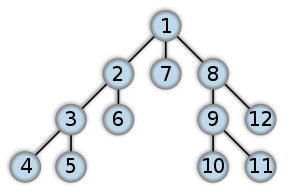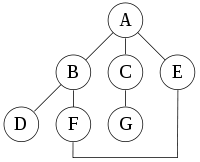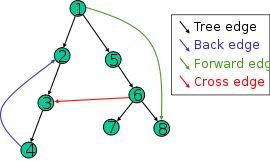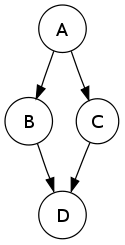# Depth-first search

Depth-first search
ClassOrder in which the nodes are visited Search algorithm Graph O( | V | + | E | ) for explicit graphs traversed without repetition, O(bd) for implicit graphs with branching factor b searched to depth d O( | V | ) if entire graph is traversed without repetition, O(longest path length searched) for implicit graphs without elimination of duplicate nodes

Depth-first search (DFS) is an algorithm for traversing or searching a tree, tree structure, or graph. One starts at the root (selecting some node as the root in the graph case) and explores as far as possible along each branch before backtracking.

A version of depth-first search was investigated in the 19th century by French mathematician Charles Pierre Trémaux as a strategy for solving mazes.

## Formal definition

Formally, DFS is an uninformed search that progresses by expanding the first child node of the search tree that appears and thus going deeper and deeper until a goal node is found, or until it hits a node that has no children. Then the search backtracks, returning to the most recent node it hasn't finished exploring. In a non-recursive implementation, all freshly expanded nodes are added to a stack for exploration.

## Properties

The time and space analysis of DFS differs according to its application area. In theoretical computer science, DFS is typically used to traverse an entire graph, and takes time O(|V| + |E|), linear in the size of the graph. In these applications it also uses space O(|V|) in the worst case to store the stack of vertices on the current search path as well as the set of already-visited vertices. Thus, in this setting, the time and space bounds are the same as for breadth-first search and the choice of which of these two algorithms to use depends less on their complexity and more on the different properties of the vertex orderings the two algorithms produce.

For applications of DFS to search problems in artificial intelligence, however, the graph to be searched is often either too large to visit in its entirety or even infinite, and DFS may suffer from non-termination when the length of a path in the search tree is infinite. Therefore, the search is only performed to a limited depth, and due to limited memory availability one typically does not use data structures that keep track of the set of all previously visited vertices. In this case, the time is still linear in the number of expanded vertices and edges (although this number is not the same as the size of the entire graph because some vertices may be searched more than once and others not at all) but the space complexity of this variant of DFS is only proportional to the depth limit, much smaller than the space needed for searching to the same depth using breadth-first search. For such applications, DFS also lends itself much better to heuristic methods of choosing a likely-looking branch. When an appropriate depth limit is not known a priori, iterative deepening depth-first search applies DFS repeatedly with a sequence of increasing limits; in the artificial intelligence mode of analysis, with a branching factor greater than one, iterative deepening increases the running time by only a constant factor over the case in which the correct depth limit is known due to the geometric growth of the number of nodes per level.

## Example

For the following graph:a depth-first search starting at A, assuming that the left edges in the shown graph are chosen before right edges, and assuming the search remembers previously-visited nodes and will not repeat them (since this is a small graph), will visit the nodes in the following order: A, B, D, F, E, C, G. The edges traversed in this search form a Trémaux tree, a structure with important applications in graph theory.

Performing the same search without remembering previously visited nodes results in visiting nodes in the order A, B, D, F, E, A, B, D, F, E, etc. forever, caught in the A, B, D, F, E cycle and never reaching C or G.

Iterative deepening is one technique to avoid this infinite loop and would reach all nodes.

## Output of a depth-first searchThe four types of edges defined by a spanning tree

The most natural result of a depth first search of a graph (if it is considered as a function rather than a procedure) is a spanning tree of the vertices reached during the search. Based on this spanning tree, the edges of the original graph can be divided into three classes: forward edges (or "discovery edges"), which point from a node of the tree to one of its descendants, back edges, which point from a node to one of its ancestors, and cross edges, which do neither. Sometimes tree edges, edges which belong to the spanning tree itself, are classified separately from forward edges. It can be shown that if the original graph is undirected then all of its edges are tree edges or back edges.

### Vertex orderings

It is also possible to use the depth-first search to linearly order the vertices of the original graph (or tree). There are three common ways of doing this:

• A preordering is a list of the vertices in the order that they were first visited by the depth-first search algorithm. This is a compact and natural way of describing the progress of the search, as was done earlier in this article. A preordering of an expression tree is the expression in Polish notation.
• A reverse postordering is the reverse of a postordering, i.e. a list of the vertices in the opposite order of their last visit. Reverse postordering is not the same as preordering. For example, when searching the directed graphbeginning at node A, one visits the nodes in sequence, to produce lists either A B D B A C A, or A C D C A B A (depending upon whether the algorithm chooses to visit B or C first). Note that repeat visits in the form of backtracking to a node, to check if it has still unvisited neighbours, are included here (even if it is found to have none). Thus the possible preorderings are A B D C and A C D B (order by node's leftmost occurrence in above list), while the possible reverse postorderings are A C B D and A B C D (order by node's rightmost occurrence in above list). Reverse postordering produces a topological sorting of any directed acyclic graph. This ordering is also useful in control flow analysis as it often represents a natural linearization of the control flow. The graph above might represent the flow of control in a code fragment like
```     if (A) then {
B
} else {
C
}
D
```
and it is natural to consider this code in the order A B C D or A C B D, but not natural to use the order A B D C or A C D B.

## Pseudocode

Input: A graph G and a vertex v of G

Output: A labeling of the edges in the connected component of v as discovery edges and back edges

```1  procedure DFS(G,v):
2      label v as explored
3      for all edges e in G.incidentEdges(v) do
4          if edge e is unexplored then
5              w ← G.opposite(v,e)
6              if vertex w is unexplored then
7                  label e as a discovery edge
8                  recursively call DFS(G,w)
9          else
10             label e as a back edge
```

## ApplicationsRandomized algorithm similar to depth-first search used in generating a maze.

Algorithms that use depth-first search as a building block include:

• Finding connected components.
• Topological sorting.
• Finding 2-(edge or vertex)-connected components.
• Finding 3-(edge or vertex)-connected components.
• Finding the bridges of a graph.
• Finding strongly connected components.
• Planarity Testing
• Solving puzzles with only one solution, such as mazes. (DFS can be adapted to find all solutions to a maze by only including nodes on the current path in the visited set.)
• Maze generation may use a randomized depth-first search.
• Finding biconnectivity in graphs.

Wikimedia Foundation. 2010.

Нужен реферат?

### Look at other dictionaries:

• Depth-First Search — Tiefensuche Tiefensuche (Depth First Search) ist in der Informatik ein Verfahren zum Suchen eines Knotens in einem Graphen. Sie zählt zu den uninformierten Suchalgorithmen. Eine Verbesserung der Tiefensuche ist die iterative Tiefensuche.… …   Deutsch Wikipedia

• Depth-first search — Algorithme de parcours en profondeur L algorithme de parcours en profondeur (ou DFS, pour Depth First Search) est le principe qui s abstrait de ce qu on connait comme la façon simple de parcourir un labyrinthe sans tourner en rond. La pédagogie… …   Wikipédia en Français

• depth-first-search — paieška į gylį statusas T sritis informatika apibrėžtis ↑Paieškos medžio apėjimo būdas, kai pirma analizuojamas tam tikro mazgo ↑pomedis, o tada – neanalizuoti to paties lygio mazgai. atitikmenys: angl. depth first search ryšiai: dar žiūrėk –… …   Enciklopedinis kompiuterijos žodynas

• depth-first search — noun an algorithm for traversing a tree or graph where one starts at the root nad explores as far as possible along each branch before backtracking …   Wiktionary

• Iterative deepening depth-first search — Graph and tree search algorithms Alpha beta pruning A* B* Beam Bellman–Ford algorithm Best first Bidirectional …   Wikipedia

• Depth-limited search — Class Search Algorithm Data structure Graph Worst case performance O( | V | + | E | ) …   Wikipedia

• Breadth-first search — Infobox Algorithm class=Search Algorithm Order in which the nodes are expanded data=Graph time=O(|V|+|E|) = O(b^d) space=O(|V|+|E|) = O(b^d) optimal=yes (for unweighted graphs) complete=yesIn graph theory, breadth first search (BFS) is a graph… …   Wikipedia

• Search algorithm — In computer science, a search algorithm, broadly speaking, is an algorithm that takes a problem as input and returns a solution to the problem, usually after evaluating a number of possible solutions. Most of the algorithms studied by computer… …   Wikipedia

• Search for HMAS Sydney and German auxiliary cruiser Kormoran — A search for the wrecks of the Australian warship HMAS Sydney and the German merchant raider Kormoran , that sank each other during World War II, ended successfully in March 2008. On 19 November 1941, the two ships fought a battle in the Indian… …   Wikipedia

• Search Engine (radio show) — Infobox Podcast title = Search Engine caption = Search Engine s Current Logo host = Jesse Brown url = http://cbc.ca/searchengine rss = http://www.cbc.ca/podcasting/includes/searchengine.xml format = Podcast, Radio genre = Technology Search Engine …   Wikipedia